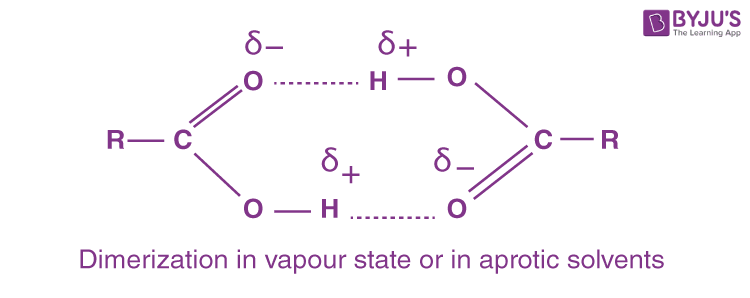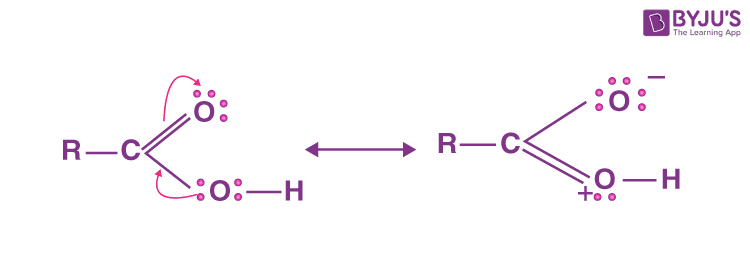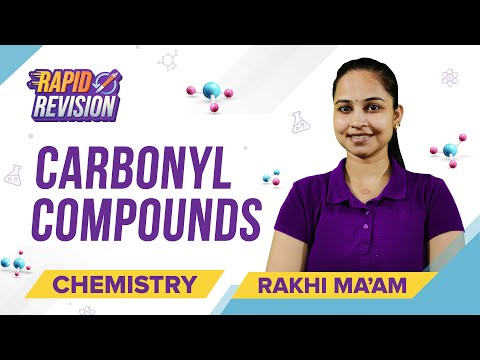Checkout JEE MAINS 2022 Question Paper Analysis : Checkout JEE MAINS 2022 Question Paper Analysis :

# Carbonyl Compounds Questions

Carbonyl Compounds include the study of the most important functional group in organic chemistry. The classes of compounds that involve the carbonyl functional group are aldehydes, ketones, carboxylic acids and their derivatives.

Definition: Carbonyl compounds involve the carbon and oxygen double bond (C=O) often called the carbonyl group.

## Carbonyl Compounds Chemistry Questions with Solutions

Q1: Why is the Collins reagent better oxidising agent than acidified K2Cr2O7 for the oxidation of the primary (1o) alcohol to form respective aldehydes?

Answer: Both the Collins reagent and the acidified K2Cr2O7 oxidise the 1o alcohol to form respective aldehydes.

RCH2-OH

$$\begin{array}{l}\xrightarrow[H_{2}SO_{4}]{K_{2}Cr_{2}O_{7}}\end{array}$$
R-CHO

RCH2-OH

$$\begin{array}{l}\xrightarrow[reagent]{Collins}\end{array}$$
R-CHO

However, if the aldehyde thus formed upon the action of acidified K2Cr2O7 is left undistilled within the same reaction mixture, the aldehyde further gets oxidised into the carboxylic acid.

R-CHO

$$\begin{array}{l}\xrightarrow[H_{2}SO_{4}]{K_{2}Cr_{2}O_{7}}\end{array}$$
R-COOH

This happens because acidified K2Cr2O7 is a strong oxidising agent and leaving the thus formed aldehyde in the solution mixture may lead to its oxidation.

While Collins reagent being the mild oxidising agent does not further oxidise the aldehyde. Collins reagent also does not attack any of the C=C double bonds present in the compound.

Q2. Write the chemical reactions of the following transformations.

1. Butanol to Butanoic acid
2. Benzyl alcohol to phenylethanoic acid

Answer: The corresponding chemical reactions for each of the following transformations are as:

1. This is a simple oxidation of alcohol to form carboxylic acid.

Since oxidation with KMnO4 in the acidic medium often results in some esters too. So, the reaction is carried by treating the alcohol with KMnO4 in neutral or in alkaline medium and therefore, treating the thus produced K-salt of the acid with dil. H2SO4 gives the desired carboxylic acid.

CH3CH2CH2CH2OH

$$\begin{array}{l}\xrightarrow[(ii.)dil.\ H_{2}SO_{4}]{(i.)KMnO_{4}/\ KOH,\ \Delta }\end{array}$$
CH3CH2CH2COOH

1. In this transformation, the alcohol functional group on the carbon attached to benzene is converted into cyanide functional group. The cyanide group under hydrolysis gives a carboxylic acid group.

C6H5CH2OH

$$\begin{array}{l}\xrightarrow[]{PBr_{3}}\end{array}$$
C6H5CH2Br
$$\begin{array}{l}\xrightarrow[]{KCN}\end{array}$$
C6H5CH2CN
$$\begin{array}{l}\xrightarrow[Hydrolysis]{H_{3}O^{+},\ \Delta }\end{array}$$
C6H5CH2COOH

Q3. Give any one test to distinguish between carboxylic acids and phenols.

Answer: When carboxylic acids are treated with NaHCO3, brisk effervescence of CO2 gas are formed. For example:

CH3COOH + NaHCO3 → CH3COONa + H2O + CO2

However, phenols do not react with NaHCO3 and hence no brisk effervescences are formed. This is called the Sodium Bicarbonate test.

Q4. The reagent used for the conversion of acid chloride to its corresponding aldehyde is ____.

Answer: In this reaction, controlled/ partial reduction of an acid chloride is done to obtain an aldehyde. Such a reduction can be brought about by the addition of H2 in the presence of Pd and some sulphur (in order to partially poison the reaction). This reaction is called Rosenmunds’ reduction in the presence of some poison such as S.

RCOCl + H2

$$\begin{array}{l}\xrightarrow[S\ or\ Quinoline]{Pd/BaSO_{4}}\end{array}$$
RCHO + HCl

Q5. Why are the carboxylic acids called fatty acids?

Answer: This is because the higher acids first discovered were obtained by the saponification of oils and fats.

Q6. Draw a scheme to convert a 1o alcohol into a carboxylic acid containing one more C-atom.

Answer: This can be done by introducing one more carbon to the 1o alcohol before converting it into an acid. Hence, the alcoholic group is replaced with a halogen (-X) by a suitable halogenating reagent. The halogen group is again replaced by a -CN group which can be further hydrolysed to form an acid containing an extra carbon than the initial alcohol.

RCH2OH

$$\begin{array}{l}\xrightarrow[-SO_{2},\ -HCl]{SOCl_{2}}\end{array}$$
RCH2Cl
$$\begin{array}{l}\xrightarrow[-KCl]{KCN}\end{array}$$
RCH2CN
$$\begin{array}{l}\xrightarrow[Hydrolysis]{H^{+}/H_{2}O}\end{array}$$
RCH2COOH

Q7. Name a reagent used to convert carboxylic acid into an alcohol.

Q8. Why do carboxylic acids exist as cyclic dimers?

Answer: Due to the polarity in the terminal O-H bonds of the carboxylic acid group, the negatively polarised Oxygen atom forms a Hydrogen bond with the positively polarised hydrogen atom of another molecule of the carboxylic acid. Due to this strong H-bonding, carboxylic acids exist as dimer in vapour phase as well as in aprotic solvents.Q9. How can an acid be converted into an ester without using any alcohol?

Answer: This can be done by using diazomethane (CH2N2).

RCOOH + CH2N2

$$\begin{array}{l}\xrightarrow[ether]{Dry}\end{array}$$
RCOOCH3 + N2

Q10. Schiff’s reagent is a

1. Magenta solution decolourised with Cl2
2. Magenta solution decolourised with sulphurous acid
3. Ammoniacal manganese sulphate solution
4. Ammoniacal cobalt chloride solution

Explanation: Schiff’s reagent is an acidic solution of fuchsin that is decolourised by sulphurous acid.

Q11. Ethanol is used for the preparation of:

1. Tincture iodine solution
2. CCl4
3. D.D.T.
4. Toluene

Explanation: Tincture Iodine is a solution containing 2-7% of the elemental iodine and Na/K-iodide dissolved in an ethanol-water mixture.

Q12. Which of the following enzymes will convert glucose into ethyl alcohol?

1. Diastase
2. Maltase
3. Zymase
4. Invertase

Explanation: Zymase catalyses the fermentation of sugars into ethanol and CO2.

Q13. The boiling point of C2H5OH is higher than C2H5SH on an account of

1. Association
2. Dissociation
3. Low mol. Mass
4. None of the above

Explanation: The C2H5OH remains in the associated form due to strong inter-molecular H-bonding which are not there in C2H5SH. Hence, C2H5OH has a higher boiling point than C2H5SH.

Q14. The aliphatic saturated ethers are often regarded as the _____ of water.

Q15. Why is the C-O bond in RCOOH shorter than that in the RC-OH?

Answer: This is because the RCOOH exhibits resonance effects.Due to the resonance effect, the C-O bond possesses some double bond character and hence have shorter bond lengths than in alcohols.

## Practise Questions on Carbonyl Compounds

Q1. Unlike acidified K2Cr2O7, why does the Collins reagent not further oxidise the aldehyde?

Q2. Give the names of the reagents used to bring about the following transformations for the formation of aldehydes.

1. Hexanol to Hexanal
2. p-Fluorotoluene to p-Fluorobenzaldehyde
3. But-2-ene to Ethanal

Q3. Arrange the boiling points of the given compounds in increasing order.

CH3CH2CH2CHO, CH3CH2CH2CH2OH, C2H5OC2H5, CH3CH2CH2CH2CH3

Q4. Why is benzaldehyde less reactive than propanal in Nucleophilic addition reactions?

Q5. Complete the following reaction.

CH3CHO + SeO2

$$\begin{array}{l}\xrightarrow[temperature]{Room}\end{array}$$

Click the PDF to check the answers for Practice Questions.

## Carbonyl Compounds## Aldehydes, Ketones and Carboxylic acids